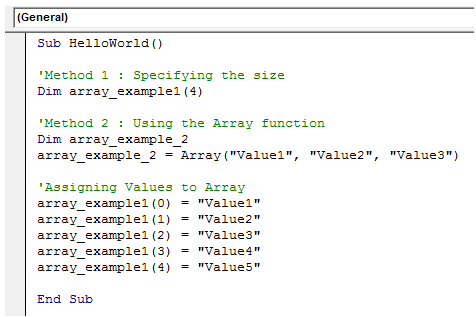## VBA arrays definition in Excel

Arrays are tantamount to variables except that, they store more than one value

Array size defines the number of values it can store at the most

Array index always starts from 0

If Array size is 4, then the index ranges from 0 to 4.

Declaration of Arrays

1. Sub Array_demo()
2. 'Method 1 : Specifying the size
3. Dim array_example1(4)
4. 'Method 2 : Using the Array function
5. Dim array_example_2
6. array_example_2 = Array("Value1", "Value2", "Value3")
7. 'Assigning Values to Array
8. array_example1(0) = "Value1"
9. array_example1(1) = "Value2"
10. array_example1(2) = "Value3"
11. array_example1(3) = "Value4"
12. array_example1(4) = "Value5"
13. End Sub

Here is how it looks like in the editor